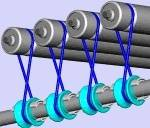# Conveyor speed

SevenToFive
I am not really buying my calculations of a gearboxes rpm for some conveyor applications. First conveyor has to travel 30 feet in 4.5 minutes with 2" rollers. If I divide 30' by 4.5minutes I get 6.667feet/minute and with a 2" diameter the gearbox should be about a 12:1 ratio.
The other is a conveyor to travel 28 feet in 20 seconds with 2" diameter rollers. IF I did this correctly I divided 28ft by 20 seconds to get 1.4ft/sec, which would give me 160rpm.

Thanks for checking my work.

30 ft in 4.5 min = 6.667 ft per min = 80” per min.
A 2” diam roller has 6.283” circumference. So roller will be at 12.733 RPM.
How you get a 12:1 gearbox ratio I do not know. It depends on the motor RPM.
A 1500 RPM motor will need a reduction of 1500 / 12.733 = 117.8 : 1 ratio.

28 feet in 20 sec = 84 ft per min = 1008” per min.
2" diameter roller has 6.283” circumference. So roller will be at 160.5 RPM.
A 1500 RPM motor will need a reduction of 1500 / 160.5 = 9.35 : 1 ratio.

•SevenToFive
Asymptotic
I am not really buying my calculations of a gearboxes rpm for some conveyor applications. First conveyor has to travel 30 feet in 4.5 minutes with 2" rollers. If I divide 30' by 4.5minutes I get 6.667feet/minute and with a 2" diameter the gearbox should be about a 12:1 ratio.
The other is a conveyor to travel 28 feet in 20 seconds with 2" diameter rollers. IF I did this correctly I divided 28ft by 20 seconds to get 1.4ft/sec, which would give me 160rpm.

Thanks for checking my work.
How is power transferred to the 2" rollers?

For instance, are they connected to a main drive shaft equipped with pulleys via heavy "rubber bands"?If so, is there a chain or belted reduction between line shaft and gearbox?

SevenToFive
30 ft in 4.5 min = 6.667 ft per min = 80” per min.
A 2” diam roller has 6.283” circumference. So roller will be at 12.733 RPM.
How you get a 12:1 gearbox ratio I do not know. It depends on the motor RPM.
A 1500 RPM motor will need a reduction of 1500 / 12.733 = 117.8 : 1 ratio.

28 feet in 20 sec = 84 ft per min = 1008” per min.
2" diameter roller has 6.283” circumference. So roller will be at 160.5 RPM.
A 1500 RPM motor will need a reduction of 1500 / 160.5 = 9.35 : 1 ratio.
I was using 1750 rpm for a motor speed. Thanks again for the help.